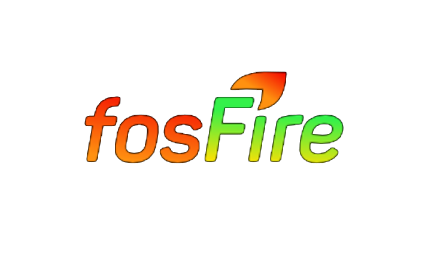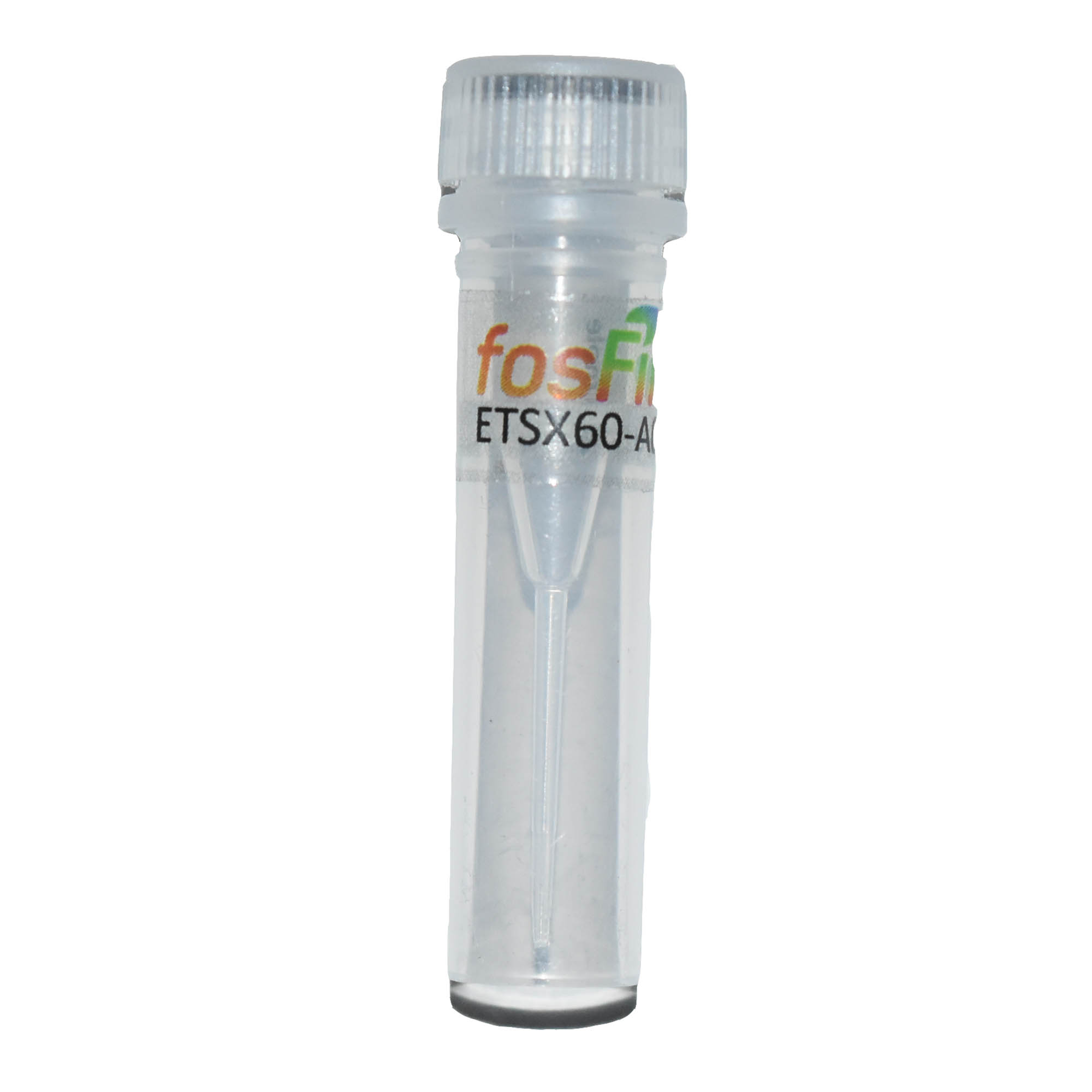# We are Excited to announce our First Mixture FosFire!# ETSX 60 - A001

fosFire luminophores Nomenclature

The product code for a luminophore is created using the following sequence of characters

ABCDxWN-nnnn

where –#nnn is the Batch CODE, # will range from A to Z and nnn from 1 to 999.

A = type of lanthanide

• E = Europium
• T = Terbium
• S = Samarium
• D = Dysprosium
• Pt = platinum
• X = other

B = ligand structure

• T = terphenyl
• B = 1,2 Dibenzyl benze (BHHBCB)
• C = Carbazole
• P = pyridine based
• D = DOTA

C = active binding group – the moiety that binds to target

• C = chlorosulfonate
• S = succinimide
• M = maleimide

D = conjugate status

• Dn = dendrimer of stage n
• Ab = antibody
• Aa = anti-antibody
• Ax = carrier of some other type of biomolecule
• X = not a conjugate

W = relative hydrophilic range – the ligand is dissolved in DMF at a concentration of 50 mg/ml and a maximum volume of 20 µL of this solution is added to 1ml of FEB solution.

• 0 = less than 100 µg/ml (hydrophobic)
• Range 1 to 8 corresponds to 100 µg/ml to 800 µg/ml (2 µL to 16 µL)
• 9 = water solubility of ligand exceeds 1 mg/ml

N = ligand status / chelated with target ion or not

• 1 = ligand is chelated with target ion
• 0 = ligand is pure and requires addition of ion

Example, BHHCT as pure ligand, has a chlorosulfonate binding group, is without added Eu3+ and was produced from the first batch A = E; B = T; C = C; D = X; W = 0; N = 0, batch code = A001 then BHHCT has product code ETCX00-A001